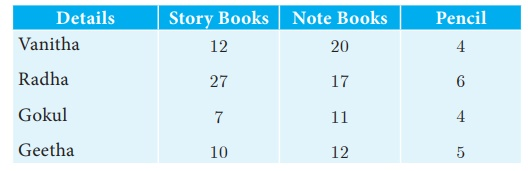Home | | Maths 10th Std | Matrices

# Matrices

A matrix is a rectangular array of elements. The horizontal arrangements are called rows and vertical arrangements are called columns.

Matrices

## Introduction

Let us consider the following information. Vanitha has 12 story books, 20 notebooks and 4 pencils. Radha has 27 story books, 17 notebooks and 6 pencils. Gokul has 7 story books, 11 notebooks and 4 pencils. Geetha has 10 story books, 12 notebooks and 5 pencils.Now we arrange this information in the tabular form as follows.Here the items possessed by four people are aligned or positioned in a rectangular array containing four horizontal and three vertical arrangements. The horizontal arrangements are called “rows” and the vertical arrangements are called “columns”. The whole rectangular arrangement is called a “Matrix”. Generally, if we arrange things in a rectangular array, we call it as “ Matrix”.

Applications of matrices are found in several scientific fields. In Physics, matrices are applied in the calculations of battery power outputs, resistor conversion of electrical energy into other forms of energy. In computer based applications, matrices play a vital role in the projection of three dimensional image into a two dimensional screen, creating a realistic seeming motions. In graphic software, Matrix Algebra is used to process linear transformations to render images. One of the most important usage of matrices are encryption of message codes. The encryption and decryption process are carried out using matrix multiplication and inverse operations. The concept of matrices is used in transmission of codes when the messages are lengthy. In Geology, matrices are used for taking seismic surveys. In Robotics, matrices are used to identify the robot movements.

## Definition

A matrix is a rectangular array of elements. The horizontal arrangements are called rows and vertical arrangements are called columns.

For example,is a matrix.

Usually capital letters such as A, B, C, X, Y, … etc., are used to represent the matrices and small letters such as a, b, c, l, m, n, a12, a13, to indicate the entries or elements of the matrices.

The following are some examples of matricesTags : Introduction, Definition , 10th Mathematics : UNIT 3 : Algebra
Study Material, Lecturing Notes, Assignment, Reference, Wiki description explanation, brief detail
10th Mathematics : UNIT 3 : Algebra : Matrices | Introduction, Definition